# Math Expanded Form Worksheets for Grade 2

To write numbers in the expanded form is to break up a number according to their place value and expand it to show the value of each individual digit. To solve the worksheet children would need to have an understanding of place values. The following is a worksheet with place value exercises. Children have to write the given numbers in expanded forms.

Place values refer to the value a digit has within a number. Place value is decided by the place a digit has in a number. Understanding place values is essential. It helps us understand the magnitude of numbers. 100 is a bigger number than 10, 12 is smaller than 120. Such things can be said only with the understanding of place values. This not only helps in discerning which number is greater instead it is also essential to understand decimal numbers.

Contents

## Math Expansion Practice WorksheetsThe worksheet has 10 sheets with eight questions in each. Children have to write the three digits number in their expanded forms.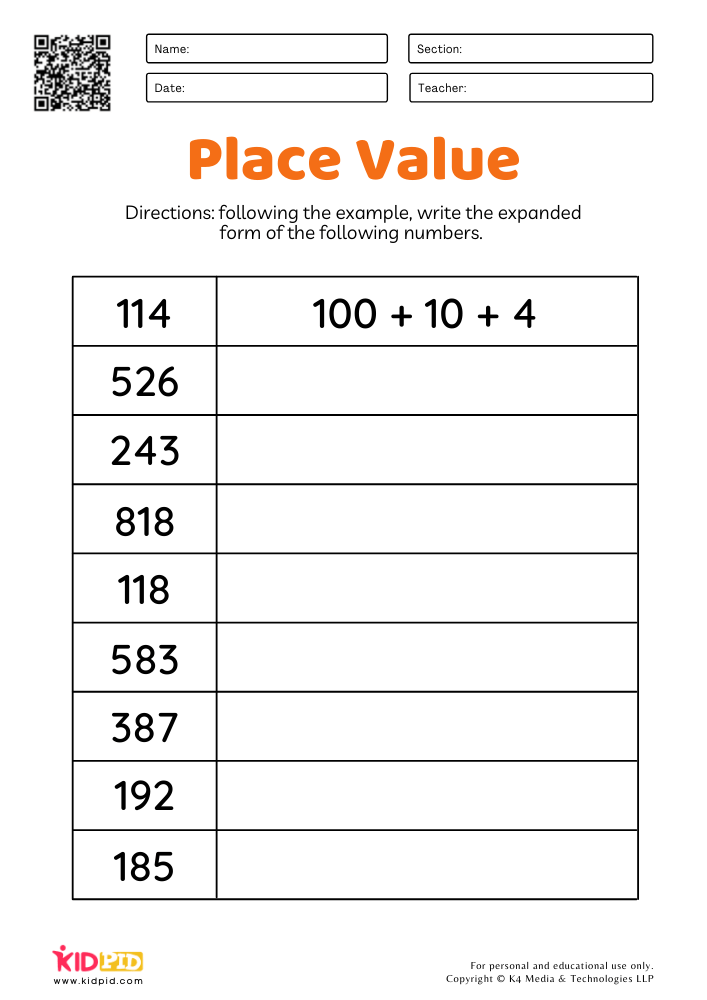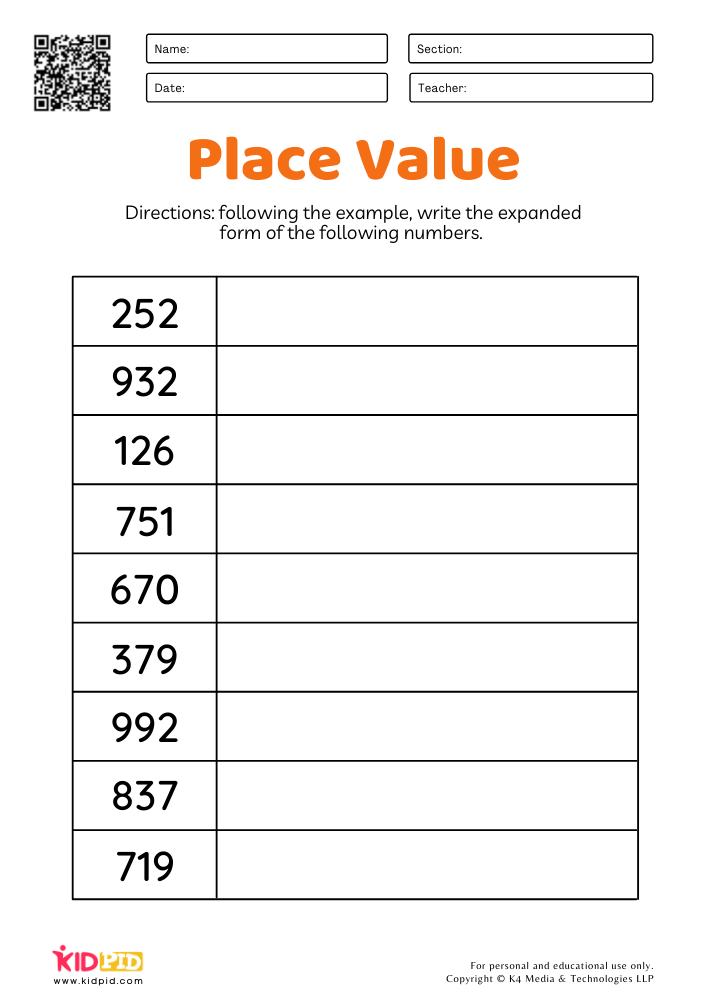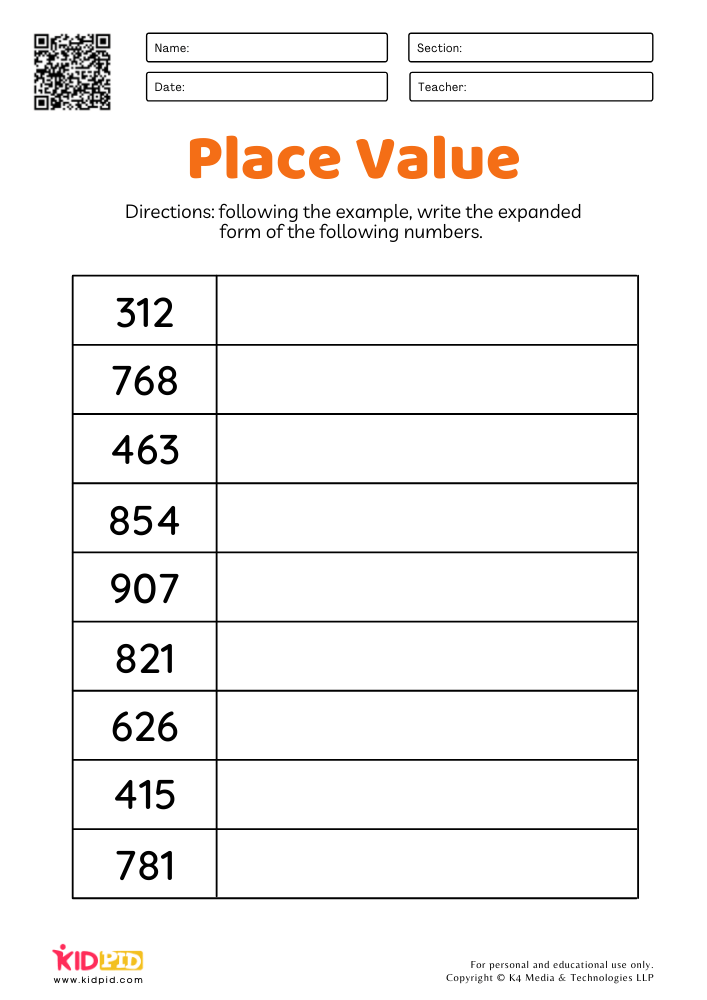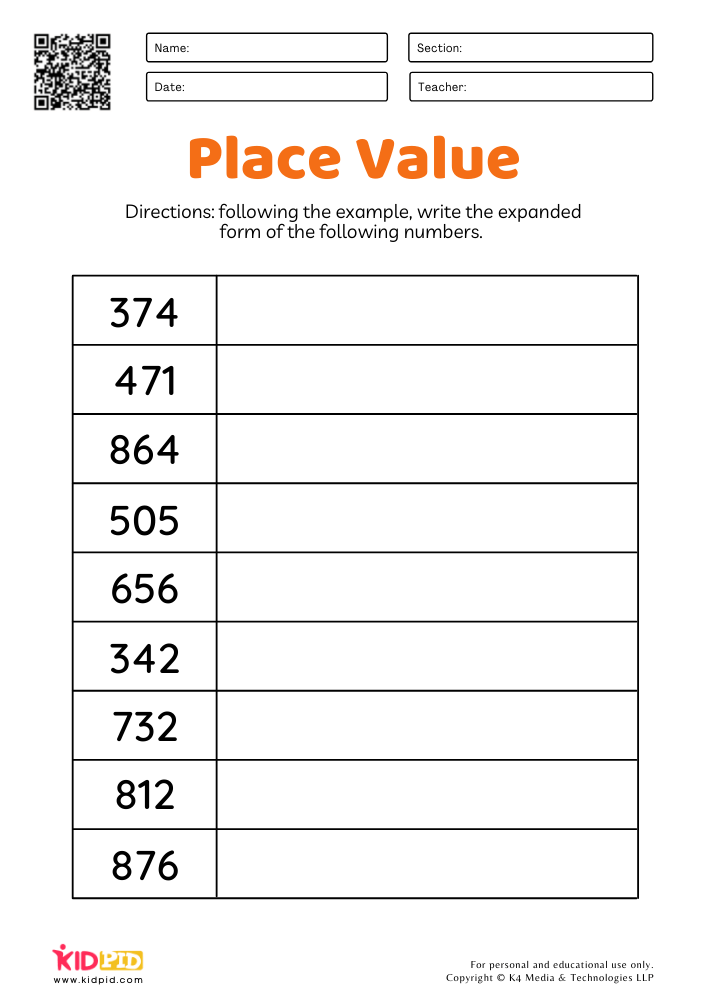Since it is such an important concept, it is essential that kids have ample practice with the exercises. The worksheet is sure to help with that. Download and print these worksheets to get started. You can distribute these sheets at once as homework or use them in classes for explaining the concept.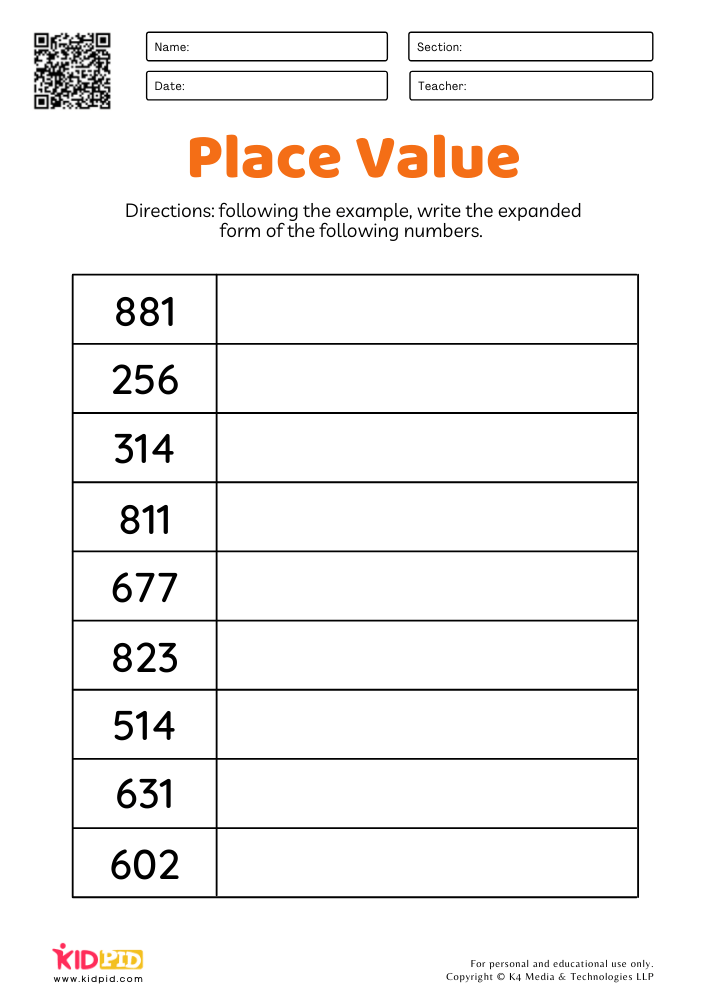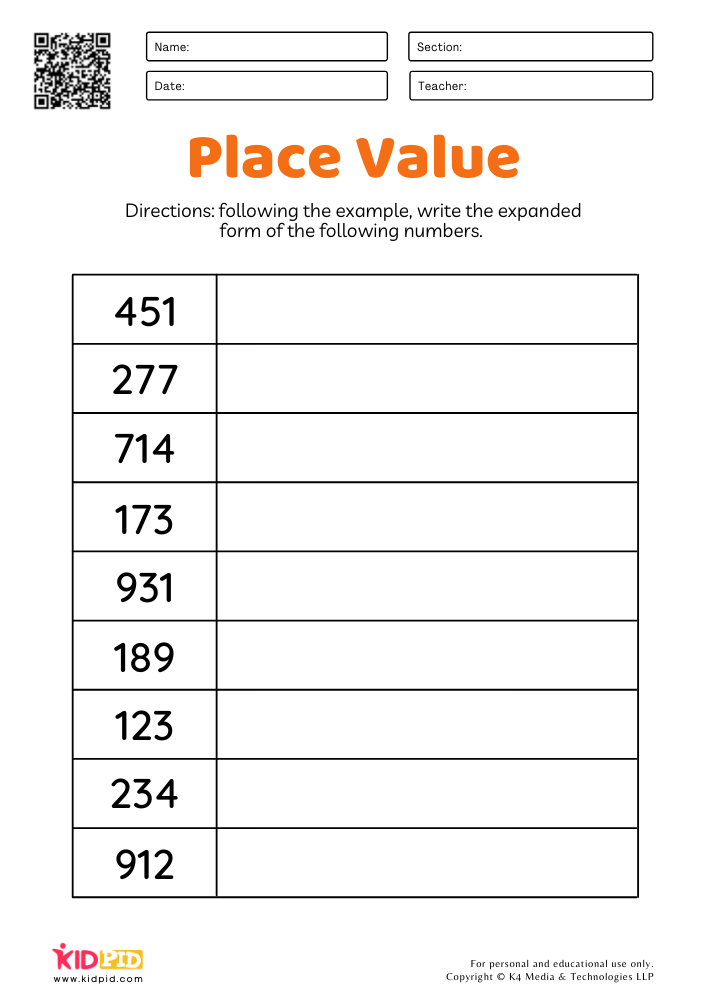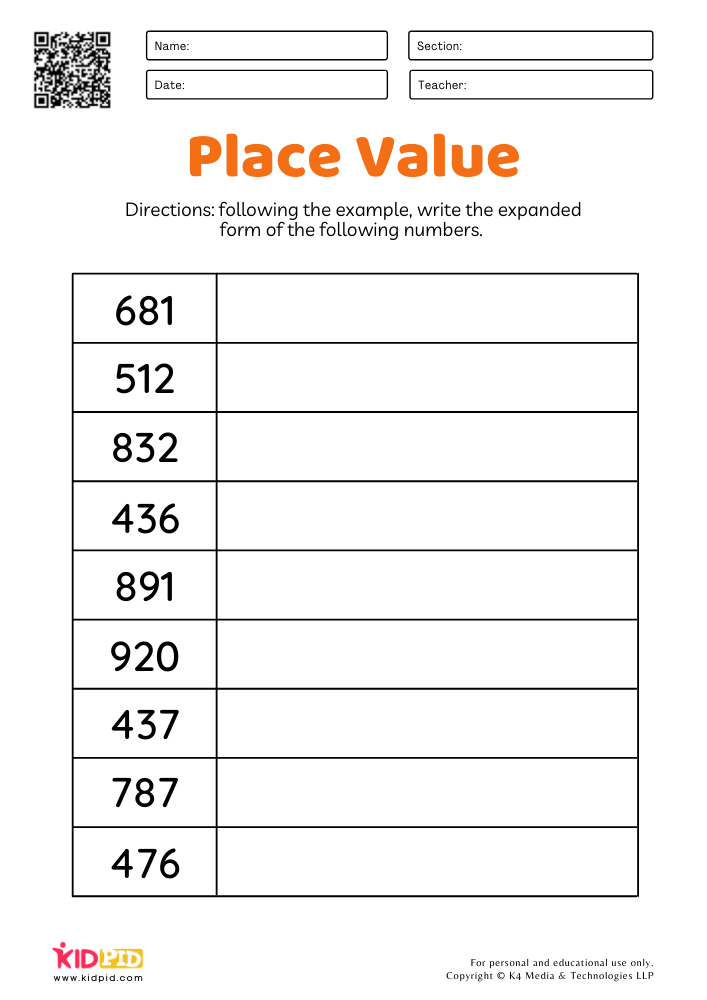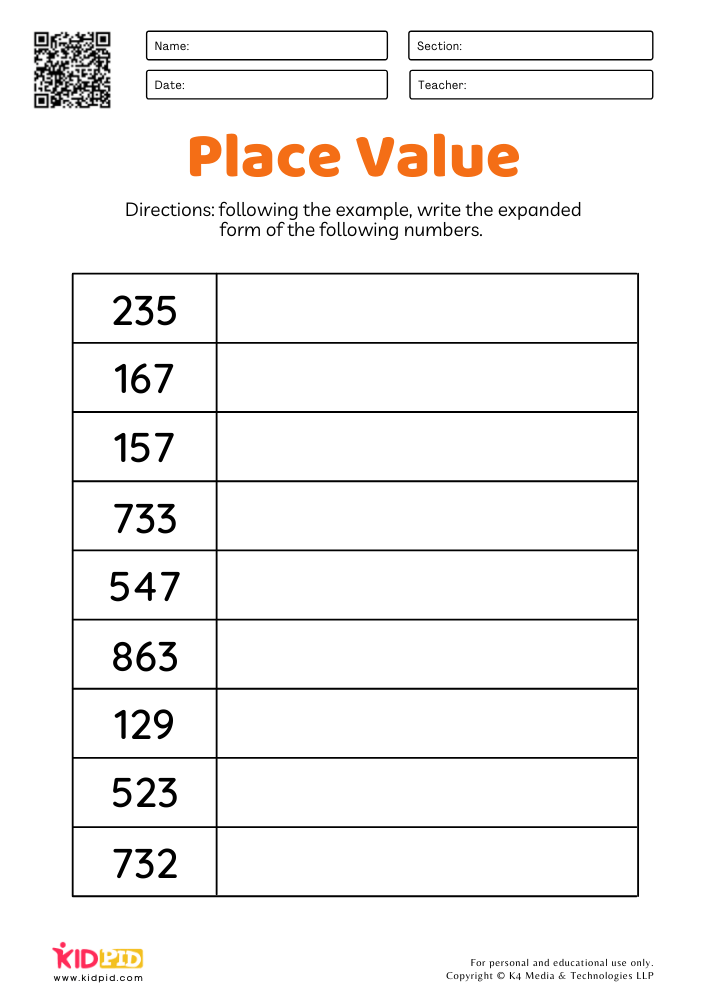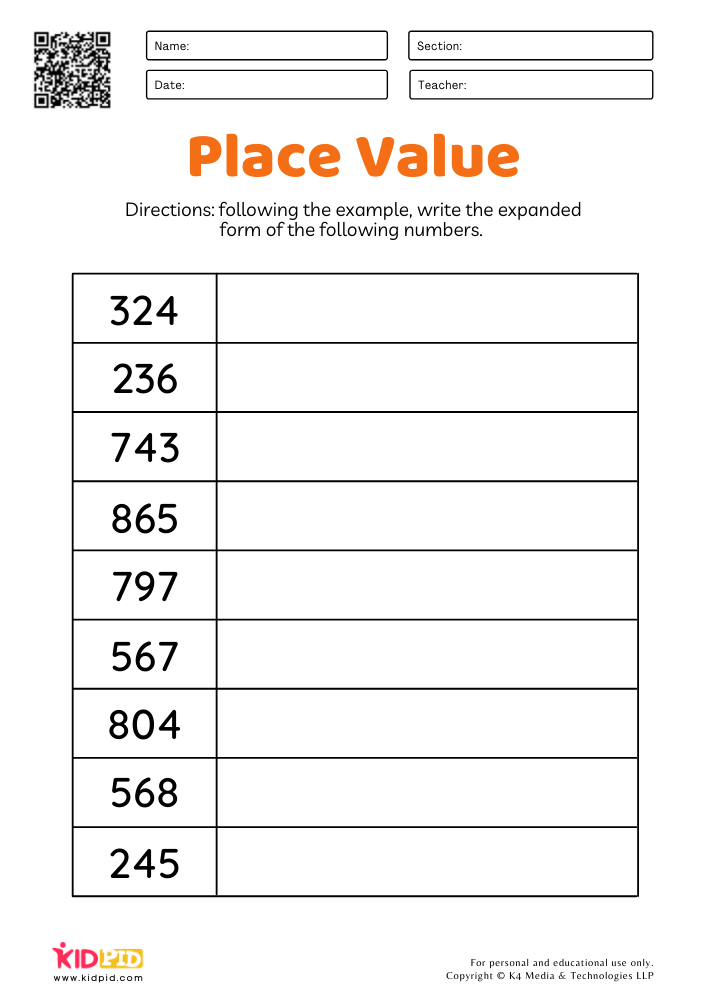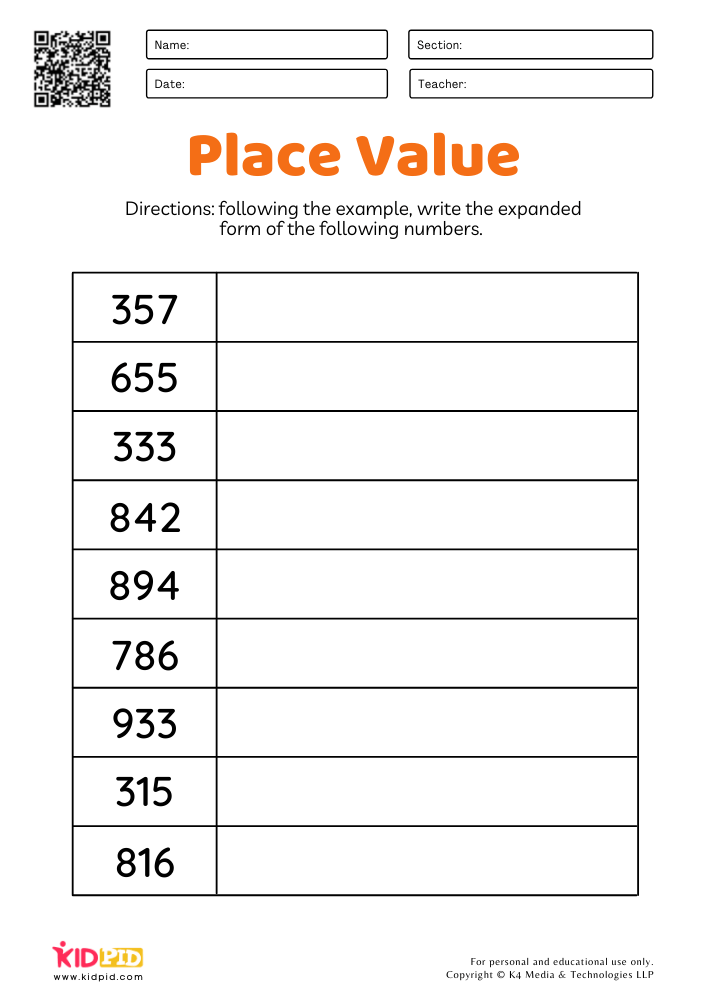Practice makes one perfect. It is important for kids to know to write numbers in expanded forms as it is with the knowledge of this that children perform arithmetic operations such as addition and subtraction which requires regrouping. For more practice, you can find several such worksheets on our website. Download now!

## FAQs

### 1. What is the purpose of Math Expanded Form Worksheets for Grade 2?

Answer: Math Expanded Form Worksheets for Grade 2 are designed to help students learn how to write numbers in the expanded form. This skill is important for students to master as it allows them to better understand the value of each digit in a number.

### 2. What types of math problems are included in Math Expanded Form Worksheets for Grade 2?

Answer: Math Expanded Form Worksheets for Grade 2 typically include problems involving addition, subtraction, multiplication, and division of numbers in expanded form.

### 3. How do students use Math Expanded Form Worksheets for Grade 2?

Answer: Students can use Math Expanded Form Worksheets for Grade 2 to practice and review their understanding of expanded form. They can use the worksheets to practice writing numbers in expanded form, as well as calculate the value of each digit in a number.

## Single-digit Addition Math Worksheets & Free Printables

We have bought single-digit addition math foundational worksheets that will help your kid in building and brushing their counting skills. In this busy world, it…

## Times Tables Multiplication Matching Card Game Flashcards

Multiplication is one of the four foundational operations in mathematics. Students are taught multiplication from a very young age since it is essential for them…

## Box Method Multiplication Worksheets for 2 Digit Numbers

We are here with box method multiplication worksheet to download and print to start a sweet mathematical journey! Your kid had learnt one-digit and two-digit…

## Greater and Less Than Flashcards

Mathematics plays an important role in the life of students. Mathematics helps to develop analytical and logical thinking in young children. For young children, it…

## Animal Multiplication Printable Worksheet for Grade 2

This animal-filled worksheet will help you improve your math abilities. The amount of spots on each animal is the same. Is your youngster able to…

+